# Excel PV function V/S FV function

In this article, we will learn about how to find Present Value using PV function and future value using FV function in Excel.
Let's understand the PV function and FV function description and syntax.

PV function returns the present value of the fixed amount paid over a period of time at a constant interest rate.

Syntax:

=PV (rate, nper, pmt, [fv], [type])

rate: Interest rate per period

nper: total no. of payment period.

pmt: amount paid each period.

fv - [optional] The present value of future payments must be entered as a negative number.

type - [optional] When payments are due. Default is 0.

FV function returns the future value of the present  amount having interest rate over a period.

Syntax:

=FV (rate, nper, pmt, [pv], [type])

rate: Interest rate per period
nper: total no. of payment period.
pmt: amount paid each period.
pv - [optional] The present value of future payments must be entered as a negative number.
type - [optional] When payments are due. Default is 0.

Present value (PV function) and Future value (FV function) are both returns amount opposite to each other. The PV function takes argument as future to return the present value whereas the FV function takes present value as argument and returns the future value for the data

Use it in example to understand more about the both functions

Here we have the amount of \$100,000 as present value considered over a period of year (12 months) at a rate of 6.5%.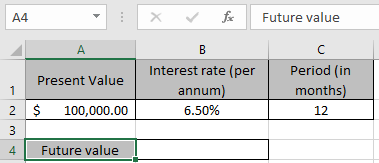And we need to find the new amount for the data after one year. We will use the FV function formula to get that new amount or future value.
Use the Formula:

= FV ( B2/12 , C2 , 0 , -A2 )

Explanation:
B2/12 : rate is divided by 12 as we are calculating interest for monthly periods.
C2 : the total time period (in months)
0 : no amount paid or received between the total period
-A2 : amount is in negative so as to get the future value amount in positive.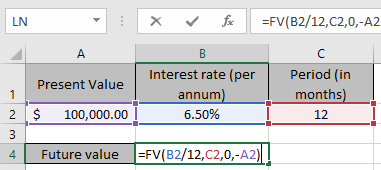Here argument to the function are given as cell reference.
Press Enter to get the result.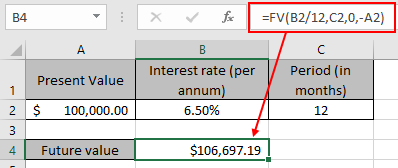This function returns a value of the amount \$106,697.19 as future value for the dataset.

Now consider the same future value for the new data set.

Here we have the amount of \$106.697.19 as the future value considered over a period of year (12 months) at a rate of 6.5%.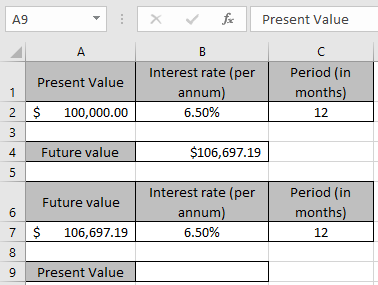And we need to find the present amount for the data before the year. We will use the FV function formula to get that present value amount.
Use the Formula:

= PV ( B7/12 , C7 , 0 , -A7 )

Explanation:
B7/12 : rate is divided by 12 as we are calculating interest for monthly periods.
C7 : the total time period (in months)
0 : no amount paid or received between the total period
-A7 : amount is in negative so as to get the present value amount in positive.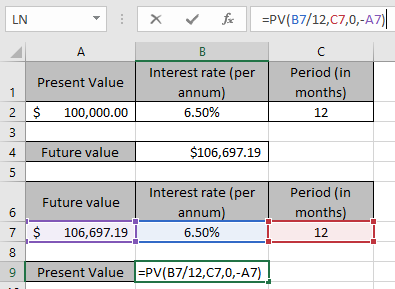Here argument to the function are given as cell reference.
Press Enter to get the result.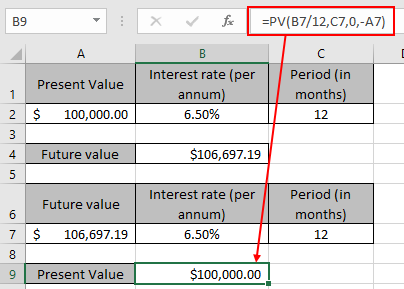This function returns a value of the amount \$100,000 as same present value for the dataset. As you can see the both functions

Hope you understood how to find the present value and future value of the amount using PV formula. Explore more articles here on Accounting. Please feel free to write your queries to us in the comment box below. We will help you.

Related Articles

How to use the PV function in excel

How to use the FV function in excel

How to use the NPV function in excel

How to use the RATE function in excel

Popular Articles

50 Excel Shortcut to Increase Your Productivity

Edit a dropdown list

If with conditional formatting

If with wildcards

Vlookup by date

Terms and Conditions of use

The applications/code on this site are distributed as is and without warranties or liability. In no event shall the owner of the copyrights, or the authors of the applications/code be liable for any loss of profit, any problems or any damage resulting from the use or evaluation of the applications/code.